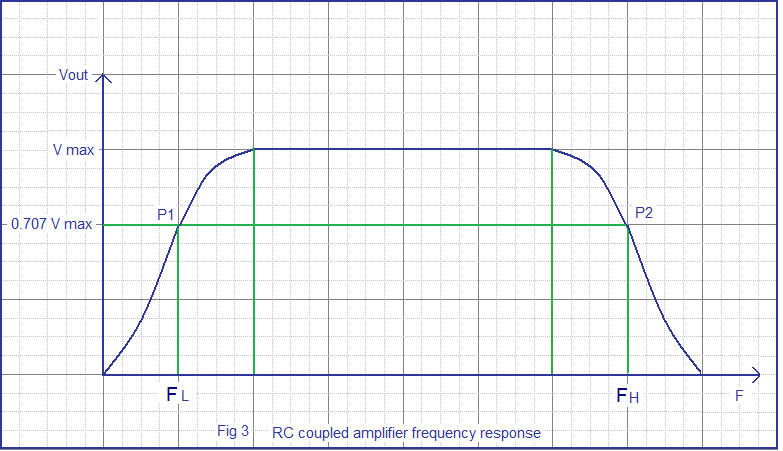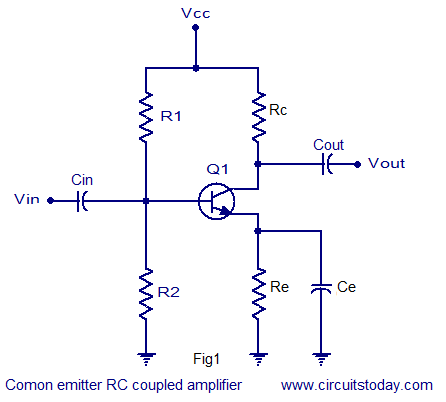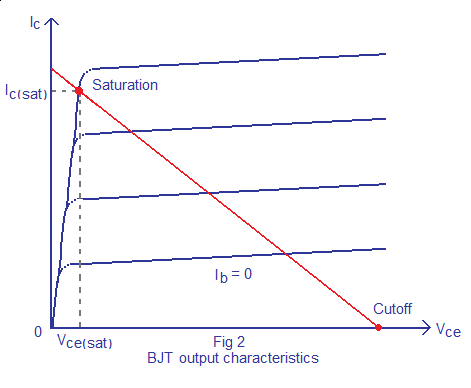## Audio

[Audio][slideshow]

Amplifier is a circuit that is used for amplifying a signal. The input signal to an amplifier will be a current or voltage and the output will be an amplified version of the input signal. An amplifier circuit which is purely based on a transistor or transistors is called a transistor amplifier. Transistors amplifiers are commonly used in applications like RF (radio frequency), audio, OFC (optic fibre communication) etc. Anyway the most common application we see in our day to day life is the usage of transistor as an audio amplifier. As you know there are three transistor configurations that are used commonly i.e. common base (CB), common collector (CC) and common emitter (CE). In common base configuration has a gain less than unity and common collector configuration (emitter follower) has a gain almost equal to unity). Common emitter follower has a gain that is positive and greater than unity. So, common emitter configuration is most commonly used in audio amplifier applications.

A good transistor amplifier must have the following parameters; high input impedance, high band width, high gain, high slew rate, high linearity, high efficiency, high stability etc. The above given parameters are explained in the next section.
Input impedance: Input impedance is the impedance seen by the input voltage source when it is connected to the input of the transistor amplifier. In order to prevent the transistor amplifier circuit from loading the input voltage source, the transistor amplifier circuit must have high input impedance.

##### Bandwidth.

The range of frequency that an amplifier can amplify properly is called the bandwidth of that particular amplifier. Usually the bandwidth is measured based on the half power points i.e. the points where the output power becomes half the peak output power in the frequency Vs output graph. In simple words, bandwidth is the difference between the lower and upper half power points. The band width of a good audio amplifier must be from 20 Hz to 20 KHz because that is the frequency range that is audible to the human ear. The frequency response of a single stage RC coupled transistor is shown in the figure below (Fig 3). Points tagged P1 and P2 are the lower and upper half power points respectively.RC coupled amplifier frequency response

##### Gain.

Gain of an amplifier is the ratio of output power to the input power. It represents how much an amplifier can amplify a given signal. Gain can be simply expressed in numbers or in decibel (dB). Gain in number is expressed by the equation G = Pout / Pin. In decibel the gain is expressed by the equation Gain in dB = 10 log (Pout / Pin). Here Pout is the power output and Pin is the power input. Gain can be also expressed in terms of output voltage / input voltage or output current / input current. Voltage gain in decibel can be expressed using the equation, Av in dB = 20 log ( Vout / Vin) and current gain in dB can be expressed using the equation Ai = 20 log (Iout / Iin).

Derivation of gain.

G = 10 log ( Pout / Pin)………(1)

Let Pout = Vout / Rout and Pin = Vin / Rin. Where Vout is the output voltage Vin is the input voltage, Pout is the output power, Pin is the input power, Rin is the input voltage and Rout is the output resistance. Substituting this in equation 1 we have
G = 10log ( Vout²/Rout) / (Vin²/Rin)………….(2)

Let Rout = Rin, then the equation 2 becomes

G = 10log ( Vout² / Vin² )
i.e.
G = 20 log ( Vout / Vin )

##### Efficiency.

Efficiency of an amplifier represents how efficiently the amplifier utilizes the power supply. In simple words it is a measure of how much power from the power supply is usefully converted to the output. Efficiency is usually expressed in percentage and the equation is ζ = (Pout/ Ps) x 100. Where ζ is the efficiency, Pout is the power output and Ps is the power drawn from the power supply.

Class A transistor amplifiers have up to 25% efficiency, Class AB has up to 55% can class C has up to 90% efficiency. Class A provides excellent signal reproduction but the efficiency is very low while Class C has high efficiency but the signal reproduction is bad. Class AB stands in between them and so it is used commonly in audio amplifier applications.

##### Stability.

Stability is the capacity of an amplifier to resist oscillations. These oscillations may be high amplitude ones masking the useful signal or very low amplitude, high frequency oscillations in the spectrum. Usually stability problems occur during high frequency operations, close to 20KHz in case of audio amplifiers. Adding a Zobel network at the output, providing negative feedback etc improves the stability.

##### Slew rate.

Slew rate of an amplifier is the maximum rate of change of output per unit time. It represents how quickly the output of an amplifier can change in response to the input. In simple words, it represents the speed of an amplifier. Slew rate is usually represented in V/μS and the equation is SR = dVo/dt.

##### Linearity.

An amplifier is said to be linear if there is a linear relationship between the input power and the output power. It represents the flatness of the gain. 100% linearity is not possible practically as the amplifiers using active devices like BJTs , JFETs or MOSFETs tend to lose gain at high frequencies due to internal parasitic capacitance. In addition to this the input DC decoupling capacitors (seen in almost all practical audio amplifier circuits) sets a lower cutoff frequency.

##### Noise.

Noise refers to unwanted and random disturbances in a signal. In simple words, it can be said to be unwanted fluctuation or frequencies present in a signal. It may be due to design flaws, component failures, external interference, due to the interaction of two or more signals present in a system, or by virtue of certain components used in the circuit.

##### Output voltage swing.

Output voltage swing is the maximum range up to which the output of an amplifier could swing. It is measured between the positive peak and negative peak and in single supply amplifiers it is measured from positive peak to the ground. It usually depends on the factors like supply voltage, biasing, and component rating.

#### Common emitter RC coupled amplifier.

The common emitter RC coupled amplifier is one of the simplest and elementary transistor amplifier that can be made. Don’t expect much boom from this little circuit, the main purpose of this circuit is pre-amplification i.e to make weak signals strong enough for further processing or amplification. If designed properly, this amplifier can provide excellent signal characteristics. The circuit diagram of a single stage common emitter RC coupled amplifier using transistor is shown in Fig1.RC coupled amplifier

Capacitor Cin is the input DC decoupling capacitor which blocks any DC component if present in the input signal from reaching the Q1 base. If any external DC voltage reaches the base of Q1, it will alter the biasing conditions and affects the performance of the amplifier.

R1 and R2 are the biasing resistors. This network provides the transistor Q1’s base with the necessary bias voltage to drive it into the active region. The region of operation where the transistor is completely switched of is called cut-off region and the region of operation where the transistor is completely switched ON (like a closed switch) is called saturation region. The region in between cut-off and saturation is called active region. Refer Fig 2 for better understanding. For a transistor amplifier to function properly, it should operate in the active region. Let us consider this simple situation where there is no biasing for the transistor. As we all know, a silicon transistor requires 0.7 volts for switch ON and surely this 0.7 V will be taken from the input audio signal by the transistor. So all parts of there input wave form with amplitude ≤ 0.7V will be absent in the output waveform. In the other hand if the transistor is given with a heavy bias at the base ,it will enter into saturation (fully ON) and behaves like a closed switch so that any further change in the base current due to the input audio signal will not cause any change in the output. The voltage across collector and emitter will be 0.2V at this condition (Vce sat = 0.2V). That is why proper biasing is required for the proper operation of a transistor amplifier.BJT output characteristics

Cout is the output DC decoupling capacitor. It prevents any DC voltage from entering into the succeeding stage from the present stage. If this capacitor is not used the output of the amplifier (Vout) will be clamped by the DC level present at the transistors collector.

Rc is the collector resistor and Re is the emitter resistor. Values of Rc and Re are so selected that 50% of Vcc gets dropped across the collector & emitter of the transistor.This is done to ensure that the operating point is positioned at the center of the load line. 40% of Vcc is dropped across Rc and 10% of Vcc is dropped across Re. A higher voltage drop across Re will reduce the output voltage swing and so it is a common practice to keep the voltage drop across Re = 10%Vcc . Ce is the emitter by-pass capacitor. At zero signal condition (i.e, no input) only the quiescent current (set by the biasing resistors R1 and R2 flows through the Re). This current is a direct current of magnitude few milli amperes and Ce does nothing. When input signal is applied, the transistor amplifies it and as a result a corresponding alternating current flows through the Re. The job of Ce is to bypass this alternating component of the emitter current. If Ce is not there , the entire emitter current will flow through Re and that causes a large voltage drop across it. This voltage drop gets added to the Vbe of the transistor and the bias settings will be altered. It reality, it is just like giving a heavy negative feedback and so it drastically reduces the gain.

#### Design of RC coupled amplifier.

The design of a single stage RC coupled amplifier is shown below.

The nominal vale of collector current Ic and hfe can be obtained from the datasheet of the transistor.

Design of Re and Ce.

Let voltage across Re; VRe = 10%Vcc ………….(1)

Voltage across Rc; VRc = 40% Vcc. ……………..(2)

The remaining 50% will drop across the collector-emitter .

From (1) and (2) Rc =0.4 (Vcc/Ic) and Re = 01(Vcc/Ic).

Design of R1 and R2.

Base current Ib = Ic/hfe.

Let Ic ≈ Ie .

Let current through R1; IR1 = 10Ib.

Also voltage across R2 ; VR2 must be equal to Vbe + VRe. From this VR2 can be found.

There fore VR1 = Vcc-VR2. Since VR1 ,VR2 and IR1 are found we can find R1 and R2 using the following equations.

R1 = VR1/IR1 and R2 = VR2/IR1.

Finding Ce.

Impedance of emitter by-pass capacitor should be one by tenth of Re.

i.e, XCe = 1/10 (Re) .

Also XCe = 1/2∏FCe.

F can be selected to be 100Hz.

From this Ce can be found.

Finding Cin.

Impedance of the input capacitor(Cin) should be one by tenth of the transistors input impedance (Rin).

i.e, XCin = 1/10 (Rin)

Rin = R1 parallel R2 parallel (1 + (hfe re))

re = 25mV/Ie.

Xcin = 1/2∏FCin.

From this Cin can be found.

Finding Cout.

Impedance of the output capacitor (Cout) must be one by tenth of the circuit’s output resistance (Rout).

i.e, XCout = 1/10 (Rout).

Rout = Rc.

XCout = 1/ 2∏FCout.

From this Cout can be found.

Setting the gain.

Introducing a suitable load resistor RL across the transistor’s collector and ground will set the gain. This is not shown in Fig1.

Expression for the voltage gain (Av) of a common emitter transistor amplifier is as follows.

Av = -(rc/re)

re = 25mV/Ie

and rc = Rc parallel RL

From this RL can be found.

[Lights][grids]